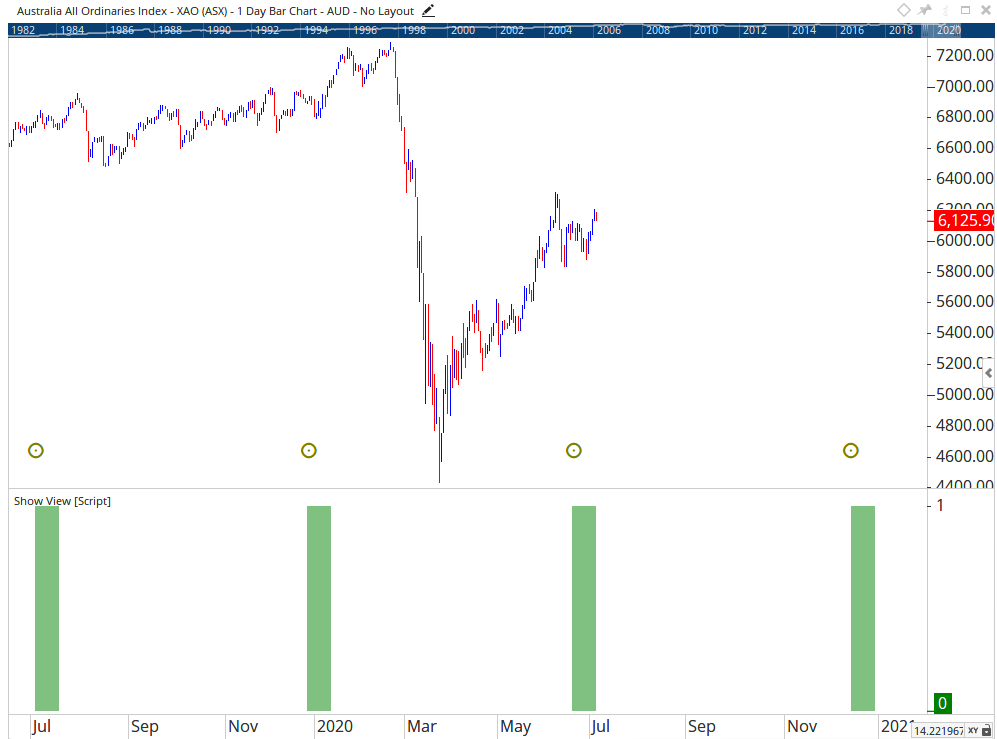# TimeSinceSignal Function

Author: Optuma Team Last updated: Jul 7, 2020 13:24

This function allows you to display the number of bars / days / weeks / months or years since a specified true condition has been met. For example this formula counts the number of trading days since the close was below the 200 day moving average:

``````//Define the true condition in variable V1
V1 = CLOSE() < MA(BARS=200);
TIMESINCESIGNAL(V1)
``````

**With the release of the Optuma v1.6 update the TimeSinceSignal() function include a new property called Signal Offset. This new property allows the function to start its count from a signal other than the very latest. In the following example there are two Show View tools setup to use the TimeSinceSignal() function.

The top one (green) is using no offset, so the count is starting from the latest trigger (Signal 0). The bottom one (blue) is using an offset of 2, and begins its count from 2 signals earlier than the last instance (Signal 2).</span>****The script used for the Blue Show View tool was:

V1 = CLOSE() CrossesAbove MA(BARS=200, STYLE=Exponential);
TIMESINCESIGNAL(V1, OFFSET=2)
**

**In the same update, the TimeSinceSignal() function has also been adjusted to support Astronomical tools, which have the ability to display data past the last bar of a chart.

In the following example the Show View is set to show zones that have occurred within 10 days of a Solar Eclipse:</span>You can see in the above example results after the last bar are being returned in the Show View.

The script used in this example is:

V1 = ECL() ;
TIMESINCESIGNAL(V1) <= 10
**

In this example, Visa hasn’t closed below its 200 day MA in 457 trading days:Note: the Show View is using Dot as the Plot Style

### To count days since a moving average crossover

This will calculate positive numbers since a 20EMA crossed above a 50SMA, and negative for a cross below:

``````// Calculate the MAs
20EMA = MA(BARS=20, STYLE=Exponential, CALC=Close);
50SMA = MA(BARS=50, CALC=Close);
// Calculate time since the MA cross
r1 = TIMESINCESIGNAL(20EMA crosses 50SMA);
// Is it a cross above?
r2 = 20EMA > 50SMA;
//Multiply by -1 to get negative numbers
r3 = r1 * -1;
//If cross is above use positive r1 value, otherwise use the negative r3 value
IF(r2 == 1,r1,r3)
``````

### To find a new high that occurred X days ago

This formula finds new 52 week highs that occurred 3 days ago:

``````V1 = HIGH() > HIGHESTHIGH(RANGE=Look Back Period, BACKTYPE=Weeks, BARS=52);
TIMESINCESIGNAL(V1) == 3
``````

For new all-time highs that occurred within the last 5 days:

``````V1 = HIGH() >= HIGHESTHIGH(RANGE=All Time);
TIMESINCESIGNAL(V1) < 5
``````